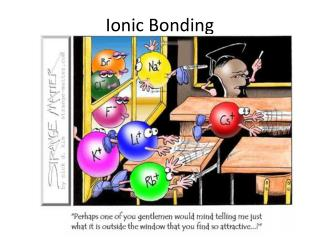DownloadDownload PresentationIonic Bonding

# Ionic Bonding

Télécharger la présentation## Ionic Bonding

- - - - - - - - - - - - - - - - - - - - - - - - - - - E N D - - - - - - - - - - - - - - - - - - - - - - - - - - -
##### Presentation Transcript

1. Ionic Bonding

2. Specification • Candidates should be able to: (a) describe the term ionic bonding as electrostatic attraction between oppositely chargedions; (b) construct ‘dot-and-cross’ diagrams, to describe ionic bonding; (c) predict ionic charge from the position of an element in the Periodic Table; (d) state the formulae for the following ions: NO3, CO32–,SO42– and NH4+;

3. Lesson Objectives • Know the difference between an ion and an atom • Describe the term ionic bond as electrostatic attraction between oppositely charged electrons • Draw cross and dot diagrams for given compounds • Draw the bonding in given polyatomic ions and state their overall charge

4. describe the term ionic bonding as electrostatic attraction between oppositely charged ions; • What is an ion? • Ions are formed when one atom transfers electrons from one atom to another • Electrons can be gained or lost • They generally do this in order to form a full outer shell

5. An aluminium atom has the electron structure 2,8,3. It needs to lose 3 electrons to become stable.

6. An oxygen atom has the electron structure 2,6. It needs to gain 2 electrons in its outer shell to become stable.

7. Lesson Objectives • Lesson Objective 1- know the difference between an atom and an ion

8. Using the whiteboards + Periodic tables predict what ions would be formed by the following • Calcium • Nitrogen • Flourine • Potassium • Bromine • Oxygen

9. Ionisation equations • Ca → Ca2+ + 2e- • N + 3e- → 3N- • Write down the equations for F,K,Br and O

10. Correct drawing of ion structures

11. Drawing Dot and Cross Diagrams Sodium Chloride

12. Electrostatic Attraction • This donation of electrons creates a positive and a negative ion • Because positives attract negatives a bond is formed • This bond is an electrostatic attraction or ionic bond

13. Lesson Objectives • Lesson Objective 2-Describe the term ionic bond as electrostatic attraction between oppositely charged electrons

14. An aluminium atom has the electron structure 2,8,3. It needs to lose 3 electrons to become stable.

15. An oxygen atom has the electron structure 2,6. It needs to gain 2 electrons in its outer shell to become stable.

16. Aluminium Oxygen To work out the number of ions of each element we need to balance the number of electrons being given and taken. 2 x 3= 6 electrons from the aluminium and 3 x 2 = 6 electrons to the oxygen 2- 3+ 2- 3+ 2- The formula is Al2O3

17. Questions Draw dot and cross diagrams for the following • LiF • Na2O • MgO • AlCl3 • MgCl2

18. Polyatomic ions • Poly-many • Atomic-atoms • E.g. hydroxide OH-(draw on board)

19. Lesson Objectives Lesson Objective 3 Draw cross and dot diagrams for given compounds

20. Extension Activity • Draw cross and dot diagrams to show the structures of the following NO3-, CO32–, SO42– and NH4+ • Predict the charge on the following polyatomic ions ClO (chlorate), PO4 (phosphate), MnO4 (permanganate) and S2O3 (thiosulphate)

21. Lesson Objective 4 Draw the bonding in given polyatomic ions and state their overall charge